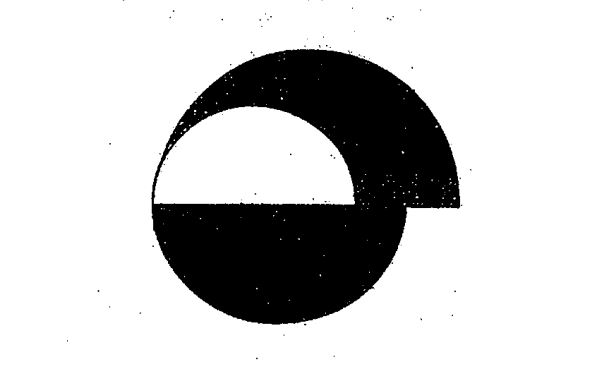# Question 1 of 79

The figure below is made up of 3 different semi-circle. The radius of the smallest semi-circle is 3cm. Using $\pi$ = 3.14, Find the area of the shaded part.A
12$\pi$
B
14$\pi$
C
16$\pi$
D
18$\pi$
E
None of the above